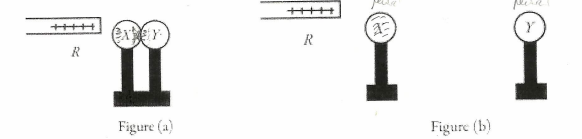# Problem: X and Y are two uncharged metal spheres on insulating stands, and are in contact with each other. A positively charged rod R is brought close to X as shown in Figure (a). Sphere Y is now moved away from X, as in Figure (b). What are the final charge states of X and Y?(a) X is positive and Y is neutral.(b) Both X and Y are neutral.(c) X is negative and Y is positive.(d) Both X and Y are negative.(e) X is neutral and Y is positive.

🤓 Based on our data, we think this question is relevant for Professor Pandit's class at USF.

###### Problem Details

X and Y are two uncharged metal spheres on insulating stands, and are in contact with each other. A positively charged rod R is brought close to X as shown in Figure (a). Sphere Y is now moved away from X, as in Figure (b). What are the final charge states of X and Y?

(a) X is positive and Y is neutral.

(b) Both X and Y are neutral.

(c) X is negative and Y is positive.

(d) Both X and Y are negative.

(e) X is neutral and Y is positive.